Do you like the article?
Share it with others -

Use new possibilities of MetaTrader 5

22 December 2017, 09:11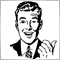11
14 492

### Introduction

Just like our real life, the market is constantly evolving. Something which did perfrectly yesterday, shows discouraging results today. However, there remain some fundamental strategies which experience some minor corrections over time, but do not change their ideological basis. One of them is “DiNapoli levels”, a strategy named after its founder. It represents stripped down realization of Fibonacci retracements

Initial points of the strategy - Fibonacci levels are 38.2% and 61.8%. For take profit points, the following are accepted: COP (Contracted Objective Point) — 61.8%, OP (Objective Point) — 100%,  XOP (Expanded Objective Point) — 161.8%. All the distances are specified in per cents from trend length (see fig. 1).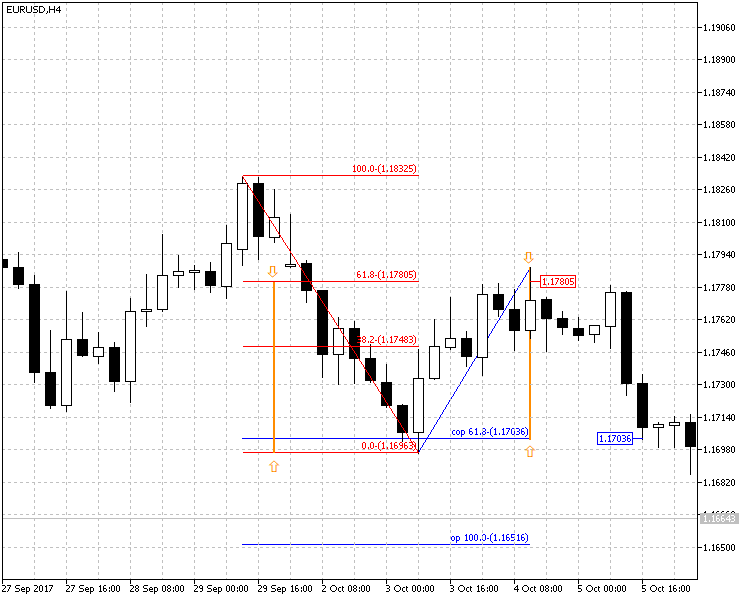Fig. 1. DiNapoli levels on currency pair EURUSD H4

At figure 1, levels are set using Fibonacci tools which are part of standard MetaTrader 5 package - Fibonacci retracements (of red color) and expansions (of blue color). Besides, the figure demonstrates how levels are set up. The vertical line marked with two arrows shows the level of 61.8%, which is taken from trend (red downward line) and is set downward from maximal rollback price, forming COP level.

There are four variants for market entering: “Bushes”, “Bonsai”, “Minesweeper A” and “Minesweeper B”. The first two are the most aggressive. Market entry by them takes place on the first correction. The last two scenarios imply opening a position on the second correction. Other differences between these variants are in placing Stop Loss level. These methods are detailed in article "DiNapoli trading system" therefore, here we will not go into details.

On the above provided figure, we just wanted to show classical picture of DiNapoli levels, the said strategy foundation. As you see, the best option to enter the market is at level 61.8% (and here you can see a red price mark), however, this level is not always reached. Therefore, some traders started to actively sell at 38.2%: this is evidenced by downward candle at 20:00. Such entries are more characteristic to agressive trading according to “Bushes” method. Following it, a trader places stop at a higher level than that, at which the position is opened. Deal closing took place at COP level (61.8%). It is marked at the figure with the blue price mark.

It looks rather simple, but in practice the described levels are not always triggered due to frequent trend variation. In order to determine the trend more definitely, the strategy author offers to use indicators in addition to levels: moving average (MA) and oscillator Stochastic. It is on this foundation, that I offer to develop a profitable strategy and to implement it in the Expert Advisor.

### Forming a strategy on standard MetaTrader 5 tools

Effective DiNapoli levels trading strategy implies joint functioning of a big number of factors. Therefore, its programming realization is rather complex and interesting. Also, there are difficulties in trading organization: according to this strategy, sometimes you have to wait for a long time for an appropriate moment to enter the market. Continuous manual monitoring is a difficult task for a trader, thus, in such trading machine factor will prevail.

I will try to maximally simply and comprehensibly explain the strategy which will serve as the basis for our Expert Advisor.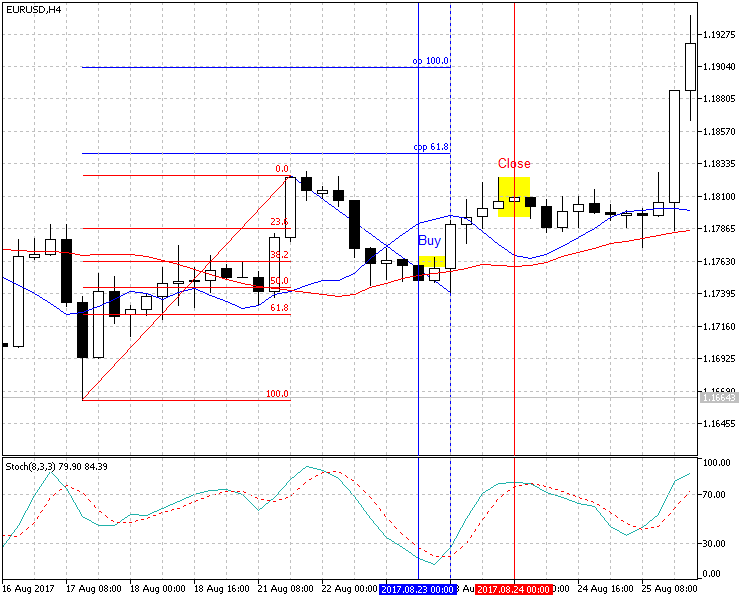Fig. 2. DiNapoli levels with auxiliary indicators EURUSD H4 (upward trend)

Figure 2 shows the chart of currency pair EURUSD H4. The following are marked on it: DiNapoli levels, indicator Stochastic (8,3,3) and two moving averages with the shift of 5 and periods of 25 (red) and 7 (blue).

Let’s start one by one. In the chart we can see an upward trend with the Grid of Fibonacci superposed over it, with levels 23.6%, 38.2%, 50%, 61.8%. Here, there are more levels than with the classical realization: practice showed that price could repel from each of them.

On rollback (correction) Fibonacci expansion is superposed: this is the way COP and OP levels occur. However, both in this case and in the strategy in general, these levels are insignificant. Here, they are calculated exclusively for setting up Take Profit (later we will see it in the code).

The net result is that the entry is possible in four points. The figure demonstrates that if catching the trend reverse in the very beginning and opening a position in point 23.6%, it will get profit; however, the drawdown will be very significant. Certainly, this will not satisfy us, therefore we will continue searching for a more reliable entry point.

• Rule one: DiNapoli level entry is possible from 23.6% to 61.8%. This is a rather wide range, that is why we should proceed to the second stage of analysis.

The following signal filter is Stochastic indicator (with parameters 8,3,3) with two levels - 30 and 70.

• Rule two: buy when the signal and main lines of Stochastic are under the level of 30; and sell when they are above the level of 70.

Now, let’s determine the trend. For doing this, use moving average (shift 5, period 25). It turns out, that buying is possible when the price is higher than trend line, while sale is possible when it is lower. But this is a quite wide range and to reduce it, let’s introduce one more moving average (shift 5, period 7). But even when using it, the range may remain too wide. In such cases its additional reduction is introduced: it is allowed to open deals only on the “upper half” of divergences, closer to the line with the period of 25.

• Rule three: buy in the second half of time interval, closer to the line with the period of 25; whereas, the line with a less time interval is placed above.
• Rule four: sell in the second half of time interval, closer to the line with the period of 25; whereas, the line with a less time interval is placed under.

Suppose, that the above listed conditions worked out and we have opened a position. Now, let’s arrange rules for its closing. For doing this, we will use the levels formed by Fibonacci expansion, as well as Stochastic indicator.

• Rule five: close a deal if the price reaches one of target DiNapoli levels (COP, OP, XOP), or Stochastic indicator will give closing signal.

In figure 2 and 3, the areas fitting these rules are shown in yellow rectangles.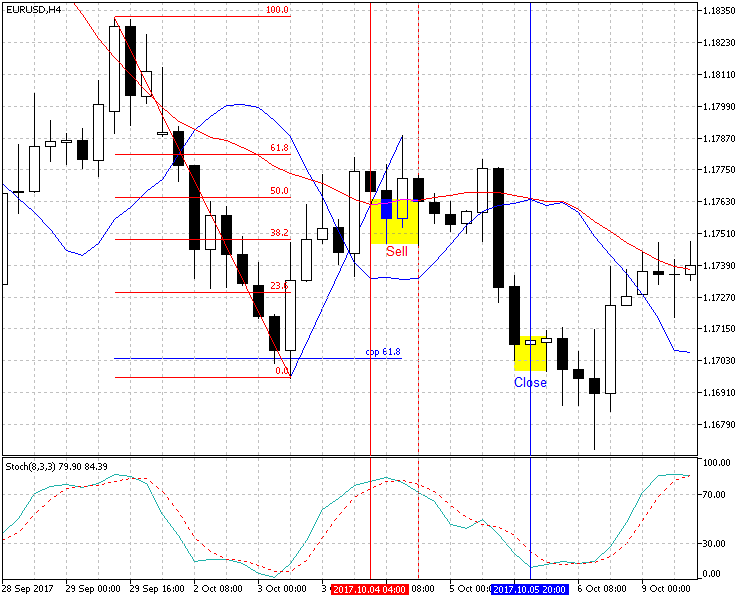Fig.3. DiNapoli levels with auxiliary indicators EURUSD H4 (downward trend)

As you can see, the resulted rules are logical and clear. Placing Take Profit or Stop Loss and minor trailing for transferring to breakeven have not yet been stipulated in them. All this will be considered below.

### Coding the Strategy

Input parameters

First, connect class CTrade (Trade.mqh). Then, establish parameters of DiNapoli levels - minimal and maximal entry level. By default, they are set as specified above: from 23.6% to 61.8%. But for different currency pairs, they may vary and be in different limits.

The following parameters are Take Profit and Stop Loss. Stop Loss is placed by levels from the peak price. In figures 2 and 3, they are shown in red. Take Profit is placed from order placing price.

That is, suppose that buy level is 50%, Stop Loss is set to 70%, Take Profit - to 70%. It means that, if assuming that 100% is 100 points, Stop Loss will be placed lower than buy price by 20 points, while Take Profit - by as much as 70 points. This must be considered when optimizing the EA.

Further, we must define trailing or passing to breakeven. According to current parameters in the code, when the price passes by 400 points, Stop Loss will shift to the profit side by 10 points.

The rest of parameters require no comments.

```//+------------------------------------------------------------------+
//|                                               Dinapoli_v1.01.mq5 |
//|                                                          Aktiniy |
//|                                            https://www.mql5.com/ |
//+------------------------------------------------------------------+
#property version   "1.01"
//--- input parameters
//--- Fibo number
input double   percent_min_enter=23.6;      // Minimal entry per cent
input double   percent_max_enter=61.8;      // Maximal entry per cent
input double   percent_for_tp=60;           // Per cent of possible Take Profit
input double   percent_for_sl=70;           // Per cent of possible Stop Loss
input int      trailing_sl=400;             // Switch to breakeven at passing through points
input int      trailing_profit=10;          // Breakeven level in points
//--- order
input long     magic_number=65758473787389; // Magic number
input double   order_volume=0.01;           // Lot size
input int      order_deviation=100;         // Deviation on position opening
//--- Stochastic
input int      stochastic_k=8;              // Indicator Stochastic “Period %К"
input int      stochastic_d=3;              // Indicator Stochastic “Slowing"
input int      stochastic_slowing=3;        // Indicator Stochastic “Period %D"
input double   stochastic_up_level=70;      // Indicator Stochastic “Signal level from top"
input double   stochastic_down_level=30;    // Indicator Stochastic "Signal level from bottom"
//--- MA RED 25 5
input int      ma_red_period=25;            // Indicator MA RED “Period"
input int      ma_red_shift=5;              // Indicator MA RED “Shift"
//--- MA BLUE 7 5
input int      ma_blue_period=7;            // Indicator MA BLUE “Period"
input int      ma_blue_shift=5;             // Indicator MA BLUE “Shift"
//--- Variable
int stochastic_handle=0;
int ma_red_handle=0;
int ma_blue_handle=0;
int fractals_handle=0;

```

In conclusion, there are determined the variables storing indicator handles and answers of signal functions to them.

Initialization

Below is a small initialization function. Here, we set up CTrade and receive indicator handles.

```//+------------------------------------------------------------------+
//| Expert initialization function                                   |
//+------------------------------------------------------------------+
int OnInit()
{
//---

stochastic_handle=iStochastic(_Symbol,_Period,stochastic_k,stochastic_d,stochastic_slowing,MODE_SMA,STO_LOWHIGH);
ma_red_handle=iMA(_Symbol,_Period,ma_red_period,ma_red_shift,MODE_SMA,PRICE_CLOSE);
ma_blue_handle=iMA(_Symbol,_Period,ma_blue_period,ma_blue_shift,MODE_SMA,PRICE_CLOSE);
fractals_handle=iFractals(_Symbol,_Period);
//---
return(INIT_SUCCEEDED);
}

```

Prior to proceeding to the main function, let us study the principle of signal functions.

Getting signal from Stochastic

```//+------------------------------------------------------------------+
//| Getting signal from Stochastic                                   |
//+------------------------------------------------------------------+
void Stochastic(char &answer)                                                                          // 0 - no signal; 1 - signal upwards; 2 - signal downwards
{
double stochastic_line_main;
double stochastic_line_signal;
int line_main=CopyBuffer(stochastic_handle,0,0,3,stochastic_line_main);
int line_signal=CopyBuffer(stochastic_handle,1,0,3,stochastic_line_signal);
if(line_main!=3 || line_signal!=3)
{Alert("Error of copy iStochastic: main line=",line_main,", signal line=",line_signal);}          // checking copied data Stochastic

if(stochastic_line_main>stochastic_up_level && stochastic_line_signal>stochastic_up_level)
if(stochastic_line_main<stochastic_down_level && stochastic_line_signal<stochastic_down_level)
}

```

This is ultimately simple: three last values of indicator are copied, the last one of them is compared with the levels preset in settings. The function passes answer in a variable.

Getting signal from indicators MA

```//+------------------------------------------------------------------+
//| Getting signal from indicators MA                                |
//+------------------------------------------------------------------+
void MA(char &answer,                                               // 0 - no signal; 1 - signal upwards; 2 - signal downwards
double &line_blue,
double &line_red,
double bid)
{
double ma_red;
double ma_blue;
int red=CopyBuffer(ma_red_handle,0,0,3,ma_red);
int blue=CopyBuffer(ma_blue_handle,0,0,3,ma_blue);
if(red!=3 || blue!=3)
{Alert("Error of copy iMA: MA red=",red,", MA blue=",blue);}   // check copied data MA red and blue

line_blue=ma_blue;
line_red=ma_red;

if(ma_blue>ma_red)                                         // supposedly the trend is directed upwards
if(ask<ma_blue)                                            // supposedly the price is in rollback position
{answer=1;}                                              // soon movement to trend direction (upwards) possible

if(ma_blue<ma_red)                                         // supposedly the trend is directed downwards
if(bid>ma_blue)                                            // supposedly the price is in rollback position
if(bid<ma_red)
{answer=2;}                                              // soon movement to trend direction (downwards) possible
}

```

Here, three last values are also copied into buffers; then, by way of comparison it is defined whether the price is between two indicator lines. Earlier, we have discussed that often the interval within which trading is possible, should be narrowed. It is due to this reason the function passes not only the answer, but also last values of two moving averages. The mechanism of trading interval narrowing will be considered later.

Receiving data from indicator Fractals

Function of trend size establishment is more complex. It is implemented on Fractals data. In the beginning of function 100 last data are copied, then checkup of upper and lower signals is performed in two cycles. Here, a test on fractal availability is realized: all the values over 10 000 are filtered off, then neighboring ones are compared searching for the biggest and the smallest first fractal from the end.

```//+------------------------------------------------------------------+
//| Receiving data from indicator Fractals                           |
//+------------------------------------------------------------------+
void Fractals(double &price_up,
double &price_down)
{
price_up=0;
price_down=0;
double fractals_up;
double fractals_down;
int up=CopyBuffer(fractals_handle,0,0,100,fractals_up);
int down=CopyBuffer(fractals_handle,1,0,100,fractals_down);
if(up!=100 || down!=100)
{Alert("Error of copy iFractals: Fractals up=",up,", Fractals down=",down);} // checking copied data Fractals up and down

double price_high=0;
double price_low=0;

for(int x=99; x>0; x--)                                                        // cycle for upper fractal
{
if(fractals_up[x]<10000)                                                    // checking fractal availability
{
if(price_high==0){price_high=fractals_up[x];}                            // initial checkup
if(price_high>fractals_up[x]){break;}                                    // if fractals are going downward stop cycle
else{price_high=fractals_up[x];}                                         // if fractals are going upward, copying
}
}

for(int x=99; x>0; x--)                                                        // repeating cycle for lower fractal
{
if(fractals_down[x]<10000)                                                  // checking fractal availability
{
if(price_low==0){price_low=fractals_down[x];}                            // initial checkup
if(price_low<fractals_down[x]){break;}                                   // if fractals are going upward, stopping cycle
else{price_low=fractals_down[x];}                                        // if fractals are going downward, copying
}
}

double price_bid=SymbolInfoDouble(_Symbol,SYMBOL_BID);                         // sell price request

if(price_high>price_ask && price_high>price_bid)                               // checking whether ask and bid prices are between the up and down price
{
price_up=price_high;                                                     // assigning values on answer
price_down=price_low;
}
}
//+------------------------------------------------------------------+

```

To make sure the signal is reliable, before the answer it is checked whether the price is within the found range. This is done because the last and probably the key fractal is formed after creation of two last candles (according to fractal formation rules).

Calculation of position opening price

The most important function is responsible for calculation of DiNapoli levels. Earlier received price maximum and minimum, as well as data on direction of the supposed trend and the last values from moving averages are passed into it. At first, all the obtained distances are transformed into points; and out of them on the basis of per cents preset in parameters sections are calculated. The sections are fractional, therefore we will normalize them.

```//+------------------------------------------------------------------+
//| Calculation of position opening price                            |
//+------------------------------------------------------------------+
void Price_for_order(double high_price,
double low_price,
double &p_enter_min,
double &p_enter_max,
double &p_tp,
double &p_sl,
char trend,                                      // 1 - upward; 2 - downward
double blue_line,
double red_line
)
{
double point_price=(high_price-low_price);
double point_enter_min=point_price*percent_min_enter/100;
double point_enter_max=point_price*percent_max_enter/100;
double point_tp=point_price*percent_for_tp/100;
double point_sl=point_price*percent_for_sl/100;
double point_ma_line=MathAbs(blue_line-red_line)/2;

point_enter_min=NormalizeDouble(point_enter_min,_Digits);
point_enter_max=NormalizeDouble(point_enter_max,_Digits);
point_ma_line=NormalizeDouble(point_ma_line,_Digits);

point_tp=NormalizeDouble(point_tp,_Digits);
point_sl=NormalizeDouble(point_sl,_Digits);

if(trend==1)                                                       // upward
{
p_enter_min=high_price-point_enter_min;
double p_ma_min=red_line+point_ma_line;
if(p_enter_min>p_ma_min)
{p_enter_min=p_ma_min;}
p_enter_max=high_price-point_enter_max;
if(p_enter_max>p_enter_min)
{p_enter_max=red_line;}

p_tp=p_enter_min+point_tp;
p_sl=high_price-point_sl;
}

if(trend==2)                                                       // downward
{
p_enter_min=low_price+point_enter_min;
double p_ma_min=red_line-point_ma_line;
if(p_enter_min<p_ma_min)
{p_enter_min=p_ma_min;}
p_enter_max=low_price+point_enter_max;
if(p_enter_max<p_enter_min)
{p_enter_max=red_line;}

p_tp=p_enter_min-point_tp;
p_sl=low_price+point_sl;
}
}

```

Further, depending on trend direction, prices are calculated and the function returns the answer.

Modification and closing of position

First, get all the data on the last position. Further, depending on order type, it gets upgraded (moves to breakeven) or closed, if Stochastic provides an opposite signal.

```//+------------------------------------------------------------------+
//| Modification and closing of position                             |
//+------------------------------------------------------------------+
double bid,
int point,
int profit,
char stochastic)
{
double sl_point=point*_Point;
double sl_profit=profit*_Point;
double tp_point=(MathAbs(tp-price))/2;

{
if(sl<price && bid>(price+sl_point))
{
sl_profit=sl_profit+price;
}
if(stochastic==2)                                 // move downward is expected
{
}
}
{
{
sl_profit=sl_profit-price;
}
if(stochastic==1)                                 // move upward is expected
{
}

}
}

```

Consolidating function

The last function consolidates all the earlier considered functions. In the beginning, all the reconciling variables are declared. Then, through indicator functions current main values of “upper strategy rules” are received. Further, division takes place depending on received answers on “upper rules”. Size of trend and prices which provide for beneficial entry to the market, are calculated. If earlier opened positions are not discovered and the price is within the calculated range, the position opens.

```//+------------------------------------------------------------------+
//| Expert tick function                                             |
//+------------------------------------------------------------------+
void OnTick()
{
//---
double price_high=0;                                           // high price for calculation on fractals
double price_low=0;                                            // low price for calculation on fractals

double price_enter_min=0;                                      // minimal entry price
double price_enter_max=0;                                      // maximal entry price
double price_tp=0;                                             // Take Profit level
double price_sl=0;                                             // Stop Loss level

double price_bid=SymbolInfoDouble(_Symbol,SYMBOL_BID);         // sell price request

double ma_blue_line=0;                                         // value of few-period МА BLUE
double ma_red_line=0;                                          // value of multi-period МА RED

if(answer_stochastic==1)                                       // move upward is expected
{
{
Fractals(price_high,price_low);
Price_for_order(price_high,price_low,price_enter_min,price_enter_max,price_tp,price_sl,1,ma_blue_line,ma_red_line);

{
if(PositionsTotal()==0)
{
}
}
}
}

{
if(answer_ma==2)                                             // move downward is expected
{
Fractals(price_high,price_low);
Price_for_order(price_high,price_low,price_enter_min,price_enter_max,price_tp,price_sl,2,ma_blue_line,ma_red_line);

if(price_bid>price_enter_min && price_bid<price_enter_max)
if(PositionsTotal()==0)
{
}
}
}

if(PositionsTotal()!=0)
{
}

}

```

In case, if open positions are already available, call position upgrade/delete function. This is the end of the code. Now, it is time we test the Expert Advisor.

### Test results

Expert Advisor Dinapoli_v1.01 was tested on various currency pairs, on timeframe H4. We used history from 2017.01.01 to 2017.11.12.

Symbol: EURUSD

Parameters:

percent_min_enter=33.6 magic_number=65758473787389 stochastic_up_level=70
percent_max_enter=56 order_volume=0.01 stochastic_down_level=30
percent_for_tp=145 order_deviation=100 ma_red_period=25
percent_for_sl=100 stochastic_k=8 ma_red_shift=5
trailing_sl=675 stochastic_d=3 ma_blue_period=7
trailing_profit=40 stochastic_slowing=3 ma_blue_shift=5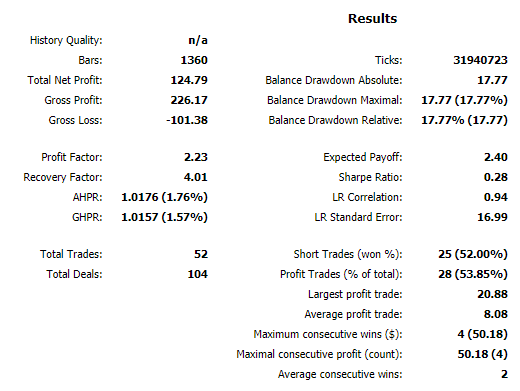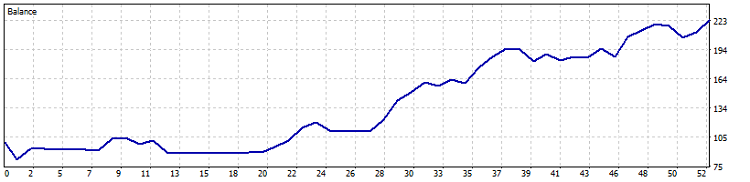Fig.4. Testing results EURUSD H4 (2017.01.01 - 2017.11.12)

Symbol: GBPUSD

Parameters:

percent_min_enter=23.6 magic_number=65758473787389 stochastic_up_level=90
percent_max_enter=50 order_volume=0.01 stochastic_down_level=30
percent_for_tp=335 order_deviation=100 ma_red_period=15
percent_for_sl=63 stochastic_k=3 ma_red_shift=5
trailing_sl=425 stochastic_d=1 ma_blue_period=4
trailing_profit=20 stochastic_slowing=4 ma_blue_shift=5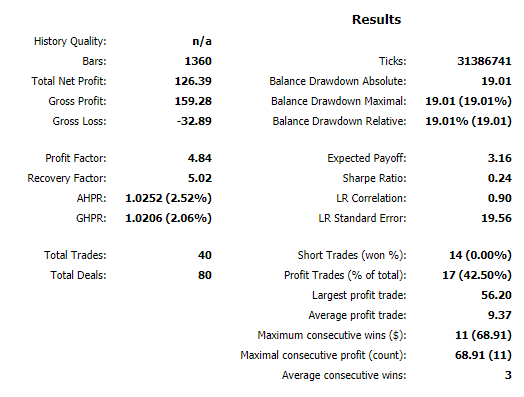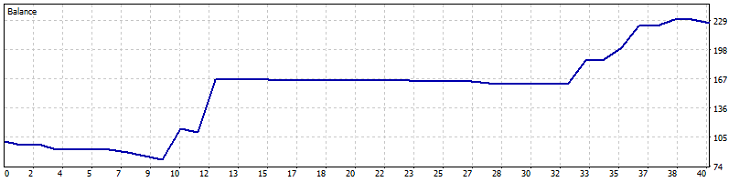Fig. 5. Testing results GBPUSD H4 (2017.01.01 - 2017.11.12)

Symbol: NZDUSD

Parameters:

percent_min_enter=31.6 magic_number=65758473787389 stochastic_up_level=60
percent_max_enter=88 order_volume=0.01 stochastic_down_level=30
percent_for_tp=45 order_deviation=100 ma_red_period=24
percent_for_sl=95 stochastic_k=10 ma_red_shift=5
trailing_sl=550 stochastic_d=2 ma_blue_period=4
trailing_profit=100 stochastic_slowing=1 ma_blue_shift=5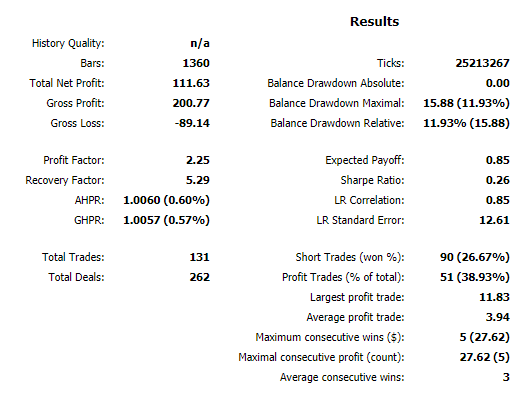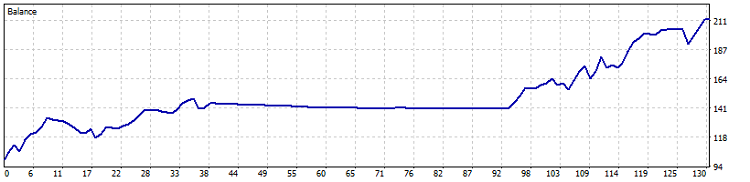Fig. 6. Testing results NZDUSD H4 (2017.01.01 - 2017.11.12)

Parameters:

percent_min_enter=23.6 magic_number=65758473787389 stochastic_up_level=60
percent_max_enter=73 order_volume=0.01 stochastic_down_level=20
percent_for_tp=315 order_deviation=100 ma_red_period=25
percent_for_sl=69 stochastic_k=10 ma_red_shift=5
trailing_sl=325 stochastic_d=1 ma_blue_period=3
trailing_profit=10 stochastic_slowing=1 ma_blue_shift=5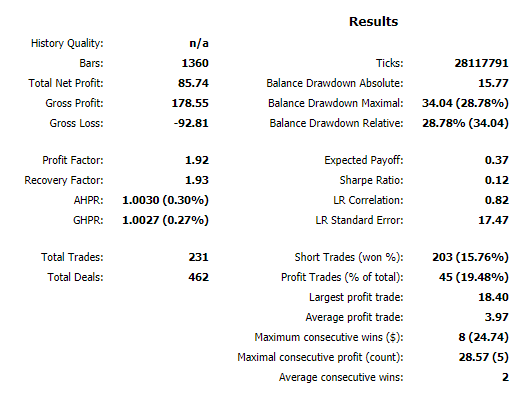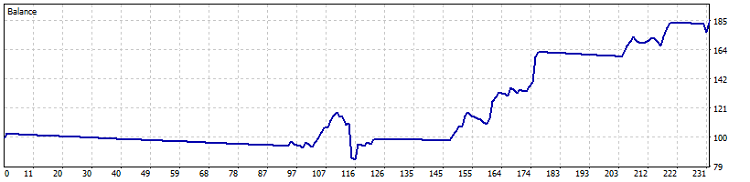Fig. 7. Testing results USDCAD H4 (2017.01.01 - 2017.11.12)

Symbol: USDCHF

Parameters:

percent_min_enter=49.6 magic_number=65758473787389 stochastic_up_level=70
percent_max_enter=69 order_volume=0.01 stochastic_down_level=40
percent_for_tp=55 order_deviation=100 ma_red_period=20
percent_for_sl=98 stochastic_k=13 ma_red_shift=5
trailing_sl=900 stochastic_d=6 ma_blue_period=12
trailing_profit=40 stochastic_slowing=3 ma_blue_shift=5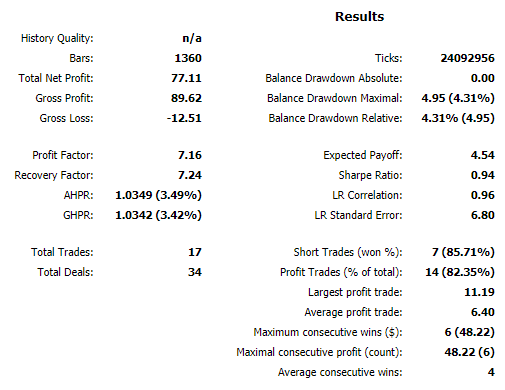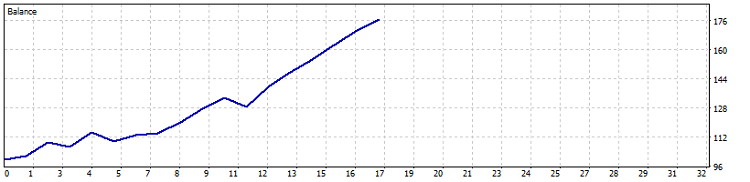Fig. 8. Testing results USDCHF H4 (2017.01.01 - 2017.11.12)

Symbol: USDJPY

Parameters:

percent_min_enter=23.6 magic_number=65758473787389 stochastic_up_level=60
percent_max_enter=50 order_volume=0.01 stochastic_down_level=20
percent_for_tp=295 order_deviation=100 ma_red_period=22
percent_for_sl=53 stochastic_k=3 ma_red_shift=5
trailing_sl=750 stochastic_d=1 ma_blue_period=5
trailing_profit=10 stochastic_slowing=1 ma_blue_shift=5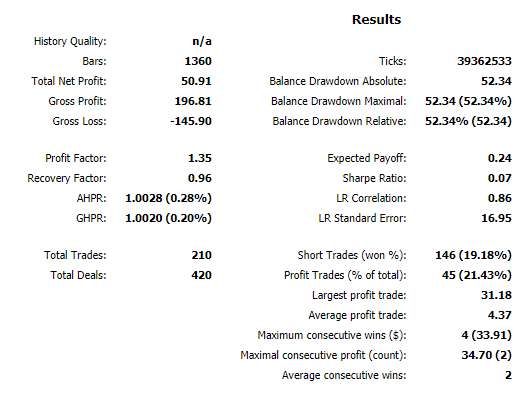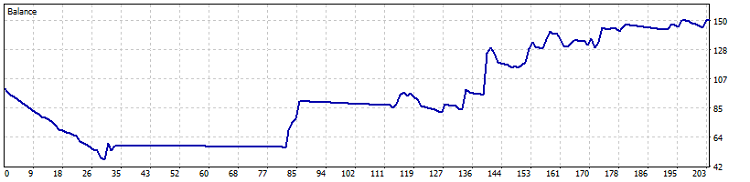Fig.9. Testing results USDJPY H4 (2017.01.01 - 2017.11.12)

Symbol: AUDUSD

Parameters:

percent_min_enter=23.6 magic_number=65758473787389 stochastic_up_level=80
percent_max_enter=81 order_volume=0.01 stochastic_down_level=40
percent_for_tp=305 order_deviation=100 ma_red_period=13
percent_for_sl=69 stochastic_k=3 ma_red_shift=5
trailing_sl=250 stochastic_d=4 ma_blue_period=1
trailing_profit=20 stochastic_slowing=4 ma_blue_shift=5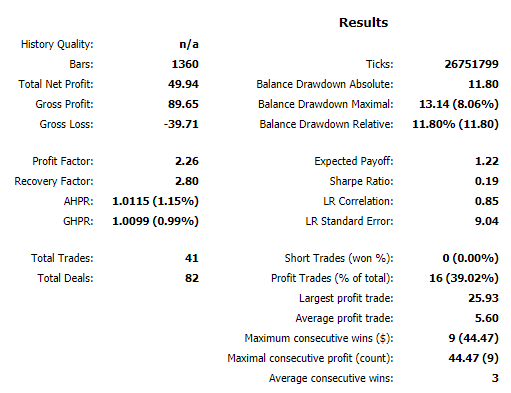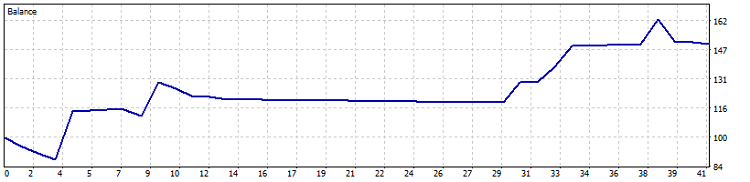Fig.10. Testing results AUDUSD H4 (2017.01.01 - 2017.11.12)

Analysis of obtained results demonstrates some instability in entries. For all the symbols, except of USHCHF, almost horizontal straight line is observed.

The reason for this is as follows: in order to reduce balance drawdown the strategy implies placement of Stop Loss as close as possible. But in such a manner requirements as to signal quality are increased. Respectively, the higher signal quality, the less entries to the market occur, but at the same time the chart price increase becomes more stable (for example, on USDCHF symbol). However, in practice we plan to receive as much profit as possible. We should make sure that the Expert Advisor will steadily minimize losses in complex market situations.

Thus, at optimization and testing you should rely upon results with a big number of entries, showed on a long period of time.

Symbol: XAUUSD

After the Expert Advisor has been tested on all the popular currency pairs, it would be good to find out how it will behave in the commodity market. Therefore, I tested XAUUSD (futures for gold to USD) and here is what I got:

Parameters:

percent_min_enter=23.6 magic_number=65758473787389 stochastic_up_level=90
percent_max_enter=50 order_volume=0.2 stochastic_down_level=10
percent_for_tp=255 order_deviation=100 ma_red_period=23
percent_for_sl=80 stochastic_k=3 ma_red_shift=5
trailing_sl=750 stochastic_d=1 ma_blue_period=6
trailing_profit=10 stochastic_slowing=1 ma_blue_shift=5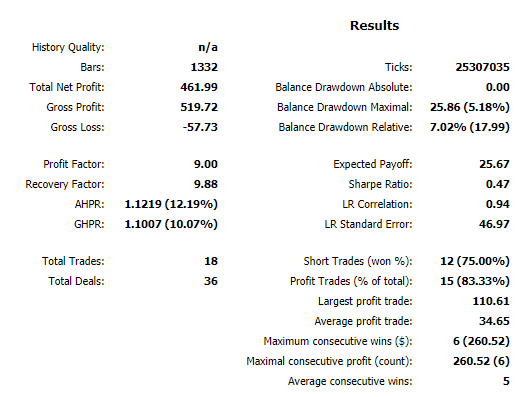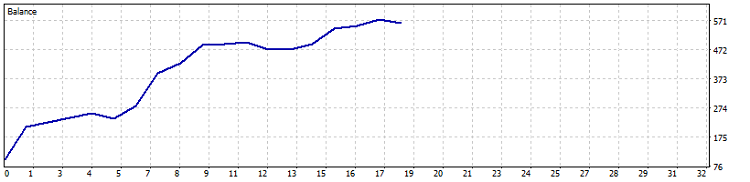Fig.11. Testing results XAUUSD H4 (2017.01.01 - 2017.11.12)

Result on gold is interesting: Expert Advisor computed entries within the accuracy of 83.3% (profitable trades) and profit constituted 461%, with drawdown not more than 40%. It means that profit by 10 times prevailed over drawdown. It is somewhat interesting index, but due to a small number of deals (totally, there are 18 trades) we cannot make serious conclusions on the basis of performed testing.

Like any other, the Expert Advisor that we developed, has its disadvantages.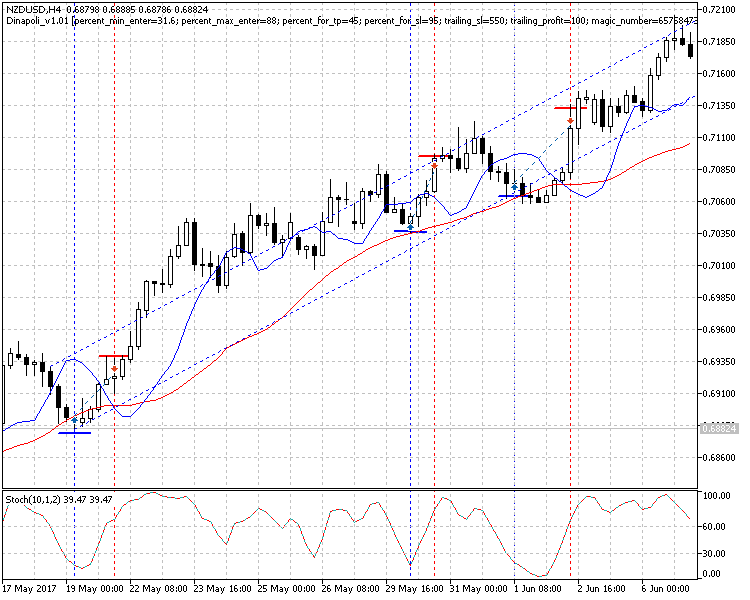Fig.12. Expert Advisor on trend (NZDUSD H4)

Figure 12 demonstrates how Expert Advisor behaves in a certain channel. It all looks rather stable, but there is the main disadvantage which is obvious: early exit from trades.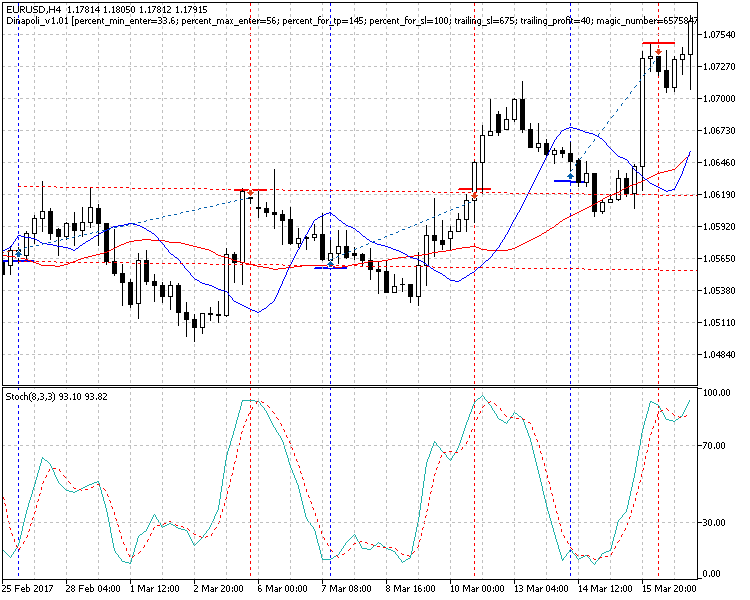Fig.13. Expert Advisor in flat (EURUSD H4)

And another “bottle neck”: Expert Advisor is not keen on working in flat. Figure 13 demonstrates that at such price movement profit is not large; also entries are calculated not very correctly.

Table of results

SymbolTradesProfit trades (%)Total net profit, \$ Max. Balance drawdown in %Expected payoff, \$PFRFSharpe
AUDUSD4116 (39.0%)49.9411.81.222.262.800.19
EURUSD5228 (53.8%)124.7917.72.402.234.010.28
GBPUSD4017 (42.5%)126.3919.03.164.845.020.24
NZDUSD13151 (38.9%)111.6311.90.852.255.290.26
USDCHF1714 (82.3%)77.114.34.547.167.240.94
USDJPY21045 (21.4%)50.8852.30.241.350.960.07
XAUUSD1815 (83.3%)461.995.1
25.679.009.880.47

From the table, you can see that results are varying, although common tendencies between different pairs are visible. With the same settings some pairs gave profit (although moderate). Finding: in order to increase profit, you should thoroughly optimize Expert Advisor settings for each currency pair.

### Conclusion

For another time we have made sure that the “old school” of traders created powerful foundation for a long time. DiNapoli level trading strategy has proved to be highly perspective; and further, I plan to continue its development. Todate, it has disadvantages: for example, at flat, Expert Advisor was rather bad. Besides, the price does not always reach COP line; and mostly, positions are effectively closed upon Stochastic.

Advantages of the developed Expert Advisor include sufficiently aggressive entries, this is particularly obvious at figure 13. Expert Advisor acts strictly according to algorithm in the situations when manually a trader, most likely, would not open a position. But, as a result, profit is achieved and drawdown is insignificant.

Attached files

# Name Type Description
1 Dinapoli_v1.01.mq5 Expert Advisor Expert Advisor realizing the strategy of using DiNapoli levels and auxiliary indicators
2 AUDUSDH4.set Expert Advisor settings file Settings used at testing and optimization for symbol AUDUSD Н4
3 EURUSDH4.set Expert Advisor settings file Settings used at testing and optimization for symbol EURUSD H4
4 GBPUSDH4.set Expert Advisor settings file Settings used at testing and optimization for symbol GBPUSD H4
5 NZDUSDH4.set Expert Advisor settings file Settings used at testing and optimization for symbol NZDUSD H4
7 USDCHFH4.set Expert Advisor settings file Settings used at testing and optimization for symbol USDCHF H4
8 USDJPYH4.set Expert Advisor settings file Settings used at testing and optimization for symbol USDJPY H4
9 XAUUSDH4.set Expert Advisor settings file Settings used at testing and optimization for symbol XAUUSD H4

Translated from Russian by MetaQuotes Software Corp.
Original article: https://www.mql5.com/ru/articles/4147

Attached files |
Dinapoli_v1.01.mq5 (29.64 KB)
AUDUSDH4.set (1.3 KB)
EURUSDH4.set (1.36 KB)
GBPUSDH4.set (1.3 KB)
NZDUSDH4.set (1.3 KB)
USDCHFH4.set (1.3 KB)
USDJPYH4.set (1.3 KB)
XAUUSDH4.set (1.3 KB)
Last comments | Go to discussion (11)| 17 Jan 2018 at 13:57

Hello,

I'm having trouble normalizing the "SL" and "TP", below I leave an image with the error "invalid stops", I know this problem is happening due to the rounding of the last digit that for the market index has to be end "0" or "5" so that "SL" and "TP" are correctly positioned without error.

I know I have the possibility to use "NormalizePrice" or "NormalizeDouble", but I could not apply this in code!

Can someone help me?

Thank you!| 28 Jan 2018 at 11:12

dear dimitry

how can I set up xauusd setup files into the adviser? does EA need any indicators except as ones which is inside MT5?

thanks for you attention and reply

Amir| 28 Jan 2018 at 11:22

my results in same period of time are different from yours. please see attachment| 31 Jan 2018 at 19:02
varshavian2:

dear dimitry

how can I set up xauusd setup files into the adviser? does EA need any indicators except as ones which is inside MT5?

thanks for you attention and reply

Amir

Hi

In the article, You can see: "Symbol: XAUUSD Parameters:"...

All the indicators used in the Expert Advisor are standard and are included in the standard MT5 kit.| 31 Jan 2018 at 19:06
varshavian2:

my results in same period of time are different from yours. please see attachment

This is possible, since on my MT5 story may not be fully loaded.
This Expert Advisor was optimized to show the possibilities of the technical analysis method itself ... And it is needed in more careful optimization ...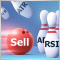Creating a new trading strategy using a technology of resolving entries into indicators

The article suggests a technology helping everyone to create custom trading strategies by assembling an individual indicator set, as well as to develop custom market entry signals.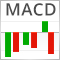Resolving entries into indicators

Different situations happen in trader’s life. Often, the history of successful trades allows us to restore a strategy, while looking at a loss history we try to develop and improve it. In both cases, we compare trades with known indicators. This article suggests methods of batch comparison of trades with a number of indicators.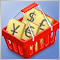Testing patterns that arise when trading currency pair baskets. Part II

We continue testing the patterns and trying the methods described in the articles about trading currency pair baskets. Let's consider in practice, whether it is possible to use the patterns of the combined WPR graph crossing the moving average. If the answer is yes, we should consider the appropriate usage methods.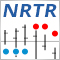The NRTR indicator and trading modules based on NRTR for the MQL5 Wizard

In this article we are going to analyze the NRTR indicator and create a trading system based on this indicator. We are going to develop a module of trading signals that can be used in creating strategies based on a combination of NRTR with additional trend confirmation indicators.